Balbharti Maharashtra State Board Class 8 Science Solutions Chapter 4 Current Electricity and Magnetism Notes, Textbook Exercise Important Questions and Answers.

## Maharashtra State Board Class 8 Science Solutions Chapter 4 Current Electricity and Magnetism

Class 8 Science Chapter 4 Current Electricity and Magnetism Textbook Questions and Answers

1. Write proper words from the following group of words in the blanks and rewrite the completed sentences:
(magnetism, 4.5V, 3.0V, gravitational attraction, potential difference, potential, higher, lower, 0V)

Question a.
Water in the waterfall flows from a higher level to the lower level because of …………… .
Water in the waterfall flows from a higher level to the lower level because of gravitational attraction.

Question b.
In an electric circuit, electrons flow from a point of potential to the point of ……….. potential.
In an electric circuit, electrons flow from a point of lower potential to the point of higher potential.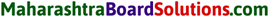Question c.
The difference between the electrostatic potential of the positive end and the negative end of an electric cell is the …………. of the cell.
The difference between the electrostatic potential of the positive end and the negative end of an electric cell is the potential difference of the cell.

Question d.
Three electric cells of potential difference 1.5 V each have been connected as a battery. The potential difference of the battery will be ……………… V.
Three electric cells of potential difference 1.5 V each have been connected as a battery. The potential difference of the battery will be 4.5 V.

Question e.
An electric current flowing in a wire creates ………………… around the wire.
An electric current flowing in a wire creates magnetism around the wire.

2. A battery is to be formed by joining 3 dry cells with connecting wires. Show how you will connect the wires by drawing a diagram.

Question a.
A battery is to be formed by joining 3 dry cells with connecting wires. Show how you will connect the wires by drawing a diagram.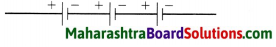3. In an electric circuit, a battery and a bulb have been connected and the battery consists of two cells of equal potential difference. If the bulb is not glowing, then which tests will you perform in order to find out the reason for the bulb not glowing?

Question a.
In an electric circuit, a battery and a bulb have been connected and the battery consists of two cells of equal potential difference. If the bulb is not glowing, then which tests will you perform in order to find out the reason for the bulb not glowing?
If you can see the filament of the bulb, check whether it is intact or broken. Check whether the cells are connected in a proper manner: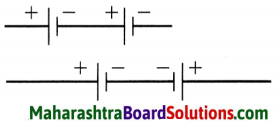Or in a wrong way:
If they are connected in a wrong way as shown above, the total potential difference will be 2V + (-2V) = zero.
Figures for reference: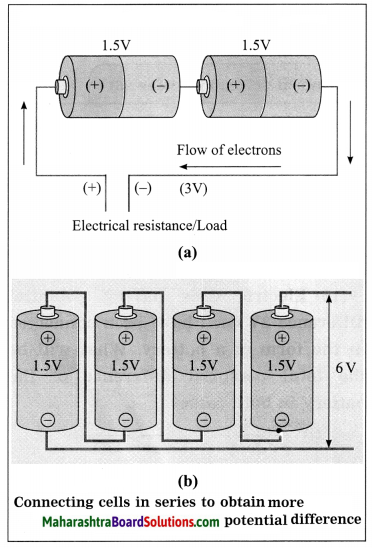4. Electric cells having potential difference 2V each have been connected in the form of a battery. What will be the total potential difference of the battery in both cases?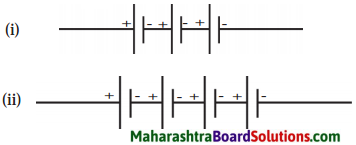Question a.
Electric cells having potential difference 2V each have been connected in the form of a battery. What will be the total potential difference of the battery in both cases?(i) 6V
(ii) 8V.
[Note: In (i), three cells are connected in series.
∴ Total potential difference = 2V + 2V + 2V = 6V.
In (ii), four cells are connected in series.
∴ Total potential difference = 2V + 2V + 2V + 2V
= 8 V]5. Describe the construction, working and usefulness of a dry cell, with the help of a diagram.
Question a.
Describe the construction, working and usefulness of a dry cell, with the help of a diagram.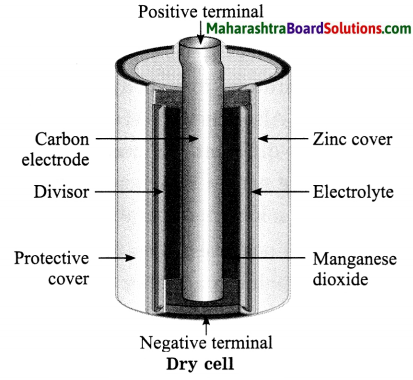Dry cell:
Take a lead dry cell and remove its outer coating. Inside you will find a whitish, metal layer. This is the zinc (Zn) metal layer. This is the negative terminal of the cell. Now, carefully break open this layer. There is another layer inside. An electrolyte is filled between these two layers.

The electrolyte contains negatively charged and positively charged ions. These are the carriers of electricity. The electrolyte is a wet pulp of zinc chloride (ZnCl2) and ammonium chloride (NH4Cl). There is a graphite rod at the centre of the cell. This is positive terminal of the cell. A paste of manganese dioxide (MnO2) is filled outside the rod. Because of the chemical reactions of all these chemicals, electrical charge is produced on the two terminals (graphite rod and zinc layer) and an electric current flows in the circuit.

Due to the wet pulp used in this cell, the chemical reaction proceeds very slowly. Hence a large electric current cannot be obtained from this. Compared to the electric cells using liquids, the shelf life of dry cells is longer. Dry cells are very convenient to use as these can be held in any direction with respect to ground and can be used in mobile instruments such as radio sets, wall clocks and torches.6. Describe the construction and working of an electric bell with the help of a diagram.

Question a.
Describe the construction and working of an electric bell with the help of a diagram.
Figure shows the construction of an electric bell and also the electric circuit. The bell consists of an electromagnet, contact screw, iron strip, metal striker and metal gong. A coil of copper wire wound around an iron piece works as an electromagnet and an iron strip along with a striker is fitted near it. A contact screw touches the strip.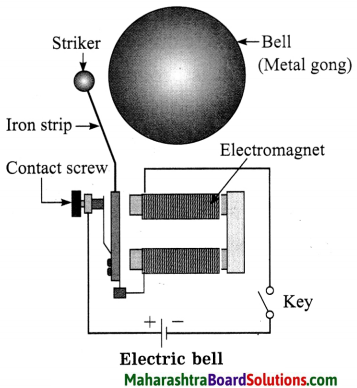The circuit is closed with the key. The current flows in the circuit when the screw is in contact with the iron strip. The current – carrying coil becomes a magnet and attracts the iron strip towards it. As a result, the metal striker hits the metal gong producing sound. At the same time, the screw loses the contact with the strip. The circuit is now incomplete.

Hence, no current flows in the circuit. Therefore, the electromagnet loses its magnetism and the electric iron strip returns to its initial condition, making contact with the screw. As the circuit is now completed, the electromagnet attracts the iron strip and the striker strikes the gong producing sound.

The action repeats itself and the bell continues to ring till the circuit is broken by opening the key in the circuit.
The working of the electric bell is thus based on the magnetic effect of electric current.Project:

Present all the activities that you performed in this chapter in Science exhibition.

Class 8 Science Chapter 4 Current Electricity and Magnetism Additional Important Questions and Answers

Rewrite the sentences after filling the blanks:

Question 1.
The SI unit of electric charge is the ……………… .
The SI unit of electric charge is the coulomb.

Question 2.
The SI unit of electric current is the ……………… .
The SI unit of electric current is the ampere.

Question 3.
The SI unit of electric potential is the ………………. .
The SI unit of electric potential is the volt.Question 4.
1 ampere = 1 coulomb per …………… .
1 ampere = 1 coulomb per second.

Question 5.
When a glass rod is rubbed on a silk cloth, the glass rod acquires …………… charge.
When a glass rod is rubbed on a silk cloth, the glass rod acquires positive charge.

Rewrite the following statements selecting the correct options:

Question 1.
The potential difference between the two electrodes of the lead-acid cell is nearly ………………. .
(a) 1 V
(b) 1.2 V
(c) 1.5 V
(d) 2 V
(d) 2 V

Question 2.
The Ni-Cd cell delivers a potential difference of …………….. .
(a) 1 V
(b) 1.2 V
(c) 1.5 V
(d) 2 V
(b) 1.2 VQuestion 3.
…………….. is a scalar quantity.
(a) Force
(b) Acceleration
(c) Velocity
(d) Electric current
(d) Electric current

Question 4.
The working of an electric bell is based on ………….. .
(a) the heating effect of electric current
(b) the chemical effect of electric current
(c) the magnetic effect of electric current
(d) the optical effect of electric current
(c) the magnetic effect of electric current

Question 5.
The coulomb is the SI unit of electric ………….. .
(a) current
(b) resistance
(c) potential
(d) charge
(d) charge

State whether the following statements are True or False:

Question 1.
The SI unit of electric potential is the ampere.
False. (The SI unit of electric potential is the volt. OR The SI unit of electric current is the ampere.)

Question 2.
In the external circuit, the conventional current flows from the positive terminal of the cell to the negative terminal of the cell.
True.

Question 3.
Very small current flows when lightning occurs.
False. (A large current flows when lightning occurs.)

Question 4.
Sensation is felt by us due to a microscopically small current flowing to the brain.
True.Question 5.
In a car battery, a current is produced by the flow of both negatively and positively charged particles.
True.

Identify the odd term:

Question 1.
Pressure exerted by a liquid, Electric current, Electric potential difference, Buoyant force.
Buoyant force. It is a vector quantity. Others are scalar quantities.

Question 2.
Electric bulb, Electric heater, Electric bell, Electric iron.
Electric bell. Its working is based on the magnetic effect of electric current. The working of other devices is based on the heating effect of electric current.

Answer the following questions in one sentence:

Question 1.
State the relation among the SI units of electric current, electric charge and time.
1 ampere = 1 coulomb per second.

Question 2.
Name the positive terminal of the dry cell.
The graphite rod at the centre is the positive terminal of the dry cell.

Question 3.
What constitutes an electric current in a metal?
A continuous flow of electrons constitutes an electric current in a metal.Question 4.
What is a battery?
A group of two or more cells connected in series to obtain more potential difference is called a battery.

Question 5.
What is a solar cell?
A solar cell is a device that converts solar energy into electrical energy.

Question 6.
State one characteristic of the Ni-Cd cell.
The Ni-Cd cell is rechargeable.

Question 7.
Give one example in which the magnetic effect of electric current is used.
The working of an electric bell is based on the magnetic effect of electric current.

Answer the following questions:

Question 1.
When do we get current electricity?
We get current electricity when charged particles such as electrons, positive ions and negative ions are made to flow in a conductor by applying electric force.

Question 2.
Explain the concept of electrostatic potential (electric potential).
A liquid flows from a higher level to a lower level. Heat flows from a body at a higher temperature to a body at a lower temperature. Also when different parts of a body are at different temperatures, heat flows from the part at a higher temperature to the part at a lower temperature.

Similarly, a positive charge (free to move) flows from a point at a higher electric level to a point at a lower electric level and a negative charge (free to move) flows from a point at a lower electric level to a point at a higher electric level. The electric level deciding the direction of flow of electric charge is called electrostatic potential (electric potential).Question 3.
What is the SI unit of electric potential?
The SI unit of electric potential is the volt (V).
[Note : This unit is named in honour of Alessandro Volta (1745-1827), Italian physicist, the inventor of the electric battery.]

Question 4.
What is potential difference?
Potential difference is the difference
between the potentials at two points. It is similar to the height of a waterfall or the temperature difference between a hot body and a cold body. Potential difference is expressed in volt.

Try this:

Take connecting copper wires and connect the ‘circuit’ as shown in Fig.(a). No current is seen to flow in the bulb. Now connect in the same ‘circuit’ a 1.5 V dry cell available in the market as shown in Fig.(b). Now it will be realized from glowing of the bulb that a current is flowing in the circuit. Electrons in the wire flow due to the potential difference between the two ends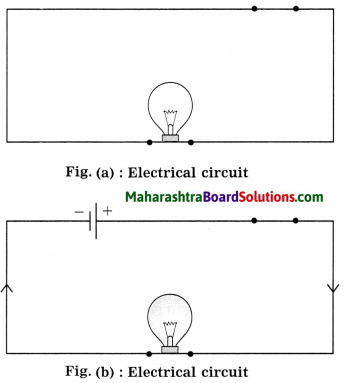In Fig.(a), there is no current as ) there is no potential difference in the absence of any cell. Current starts flowing in the circuit as soon as the potential difference is applied. The unit of potential difference in SI system is the volt (V). We will learn about it in the next standard.Question 5.
What is electric circuit or electrical circuit?
A continuous path consisting of conducting wires, a switch or a plug key and other resistances (for example, resistance of an electric bulb) between the terminals of a cell (or a battery) along which an electric current flows is called an electric circuit.

Question 6.
What is electric current?
Electric current is the electric charge flowing through a conductor, such as a metal wire, per unit time.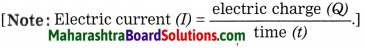Question 7.
What is one ampere?
If one coulomb of charge is passing through any cross section of a conductor in one second, the amount of current flowing through it is called one ampere.
[Note: 1 ampere = 1 coulomb per second OR 1A = 1 C/s. The coulomb is named in honour of Charles Augustin de Coulomb (1736 – 1806), French physicist. The ampere is named in honour of Andre Ampere (1175 – 1836), French physicist and mathematician.]

Question a.
How can we measure water flow emerging from a pipe? We can find it from the amount of water (litres) coming out in a specific time period. How then is the electric current measured?
Electric current, I = Q/t. In principle, if we can measure the quantity of charge (Q) flowing through a cross section of a conductor in time t, we can determine the current (i) using this formula (for a steady current). In practice, this is not done. Usually, electric current is measured using the magnetic effect of electric current. One can also use the heating effect and chemical effect of electric current for measurement of electric current.Question 8.
What is an electric cell? What is its main function?
An electric cell is a general device used to produce a uniform flow of charges in a circuit. Its main function is to maintain a constant potential difference between its two terminals.

Question 9.
Name ten devices in which electric cells are used.
Electric cells are used in radio sets, wall clocks, wrist watches, torches, toys, mobile phones, cars, invertors, remote controls, ships, submarines, satellites, etc.

Question 10.
Draw a neat labelled diagram to show the design of the lead-acid cell and explain its principle of working.
The lead-acid cell contains two electrodes, Pb and PbO2, dipped in dilute H2SO4. Chemical reaction between the substances in the cell produces electric charges on the electrodes. PbO2 carries a positive charge and Pb carries a negative charge. The potential difference between the electrodes is nearly 2V.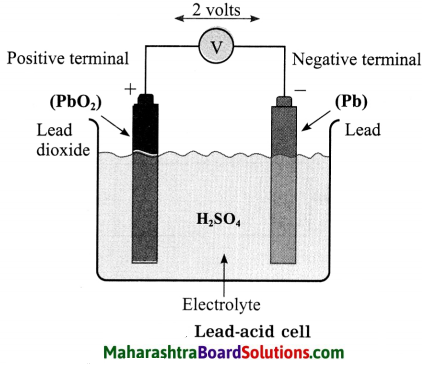If a load, such as a bulb, is connected externally between the two electrodes, an electric current flows through it. It can deliver a large current. This cell can be recharged after getting electrically discharged.

Question 11.
State applications of the lead-acid cell.
Lead-acid cells are connected in series to obtain higher potential difference. The lead-acid battery is used in cars, trucks, motorcycles and uninterrupted power supplies (UPS).Question 12.
What is the potential difference delivered by the Ni-Cd cell?
The potential difference delivered by the Ni-Cd cell is (about) 1.2 V.
[Note: In this cell, nickel oxide is the positive electrode and cadmium is the negative electrode. It is often used as a dry cell and it can be recharged. These cells are used in some portable machines that run on electricity like a drilling machine or a gardening tool.]

Question 13.
Draw a neat labelled diagram of a simple electric circuit containing a cell, an electric bulb and a plug key. What happens when the key is (i) open (ii) closed?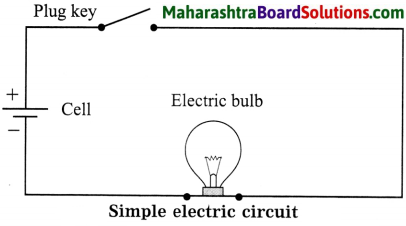(i) When the key is open, there is no current in the circuit. Hence, the bulb does not glow.
(ii) When the key is closed, a current flows in the circuit. Hence, the bulb glows.

Figure for reference:
Here, six cells can be connected in series to obtain more potential difference.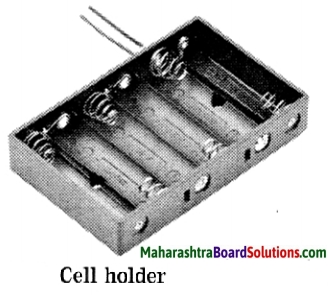Research:
Lithium ion cells are used in modern equipment for example, a smart phone, laptop, etc. These cells can be recharged. More electrical energy can be stored in these cells as compared to that in Ni-Cd cells.

Question 14.
See Fig. What is the potential difference between A and B if each cell delivers a potential difference of 2V?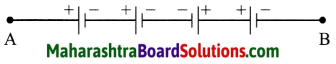Potential difference between A and B
= 2V + 2V + (-2V) + 2V = 4V.

Use your brain power!

Question a.
You must have seen the car battery available in the market. It is called a battery and not a cell. Why?
In a car battery, a number of cells are connected in series to obtain more potential difference.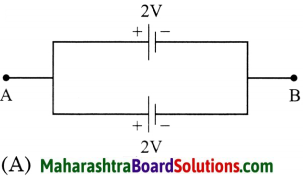Here, two identical cells are connected in parallel. The potential difference between A and B is 2V. This arrangement can be used for a longer time compared to a single cell.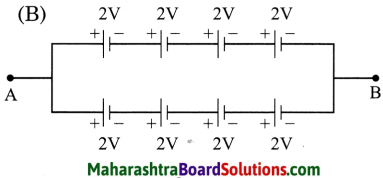Series and parallel arrangement for higher potential difference and longer life.
Here, the potential difference between A and B is 8V.

Try this:

Magnetic effects of electric current :
Activity 1 :
Take the inside tray of a used up matchbox. Place a small magnetic needle inside the tray. Now take a long connecting wire and wind it around the tray. Complete the electric circuit by connecting in it, this wire, electric cell, plug key and a bulb Figure.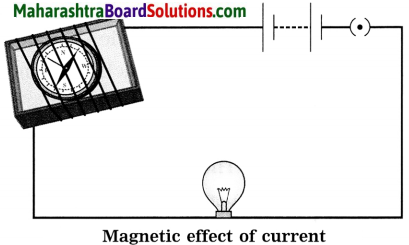Mark the position of the magnetic needle. Take a bar magnet near to the magnetic needle.
1. What do you observe? Keep looking at the needle and close the plug key. The bulb will light up, and you will realize that the current has started flowing.
2. Does the magnetic needle change its position? Now open the plug key.
3. Does the magnetic needle come back to the original position?
4. What will you conclude from this experiment?
You know that a magnetic needle is indeed a magnet. You have seen that the magnetic needle changes its direction when a bar magnet is taken near the magnetic needle. Also, you have observed that the magnetic needle changes its direction when a current starts flowing in the circuit. This means that magnetic field is created when an electric current flows in a wire. Hans Christian Oersted made this observation first. Briefly we can say that when an electric current passes through a wire, a magnetic field is produced around that wire.

1. The magnetic needle is deflected.
2. Yes.
3. Yes.
4. The electric current produces a magnetic field around a current-carrying conductor.

Activity 2 :
Take a metre long flexible copper wire having resistive coating and wind it tightly on a long iron screw. Connect the two ends of the wire in a circuit as shown in the Figure. Also connect an electric cell and a plug key in the circuit. Keep 2-4 iron pins/small nails near the screw. Now start the current in the circuit by plugging the key. It will be noticed that the pins/nails have stuck to the tip of the screw. Will the pins/nails continue to stick when the plug key is opened?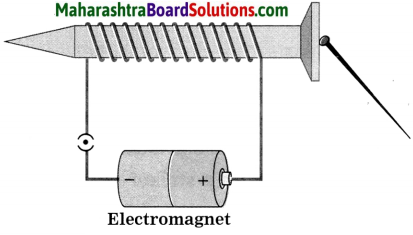No.
When the electric current flows in the wire, magnetism is produced in the coil around the screw and because of that the screw also attains magnetism. As soon as the current is stopped, this magnetism vanishes. The system of the coil and the screw is called an electromagnet. You have seen various uses of the electromagnet in the sixth standard. Electromagnets are used to produce a strong magnetic field useful in scientific research.Question 15.
What is an electromagnet? State ! its applications.
If a coil is wound around an iron screw (or an iron rod) and a current is passed through the coil with a cell (or a battery), the screw behaves as a magnet as long as there is current in the coil. The i system of the coil and the screw is called an electromagnet. It is an example of magnetic effect of electric current.
Applications: Electric bell, cranes for moving heavy loads from one place to another, toys that run on electric cells.

Question 16.
How can the strength of an electromagnet be increased?